# NCERT Solutions For Class 11 Physics Oscillations## myCBSEguide App

CBSE, NCERT, JEE Main, NEET-UG, NDA, Exam Papers, Question Bank, NCERT Solutions, Exemplars, Revision Notes, Free Videos, MCQ Tests & more.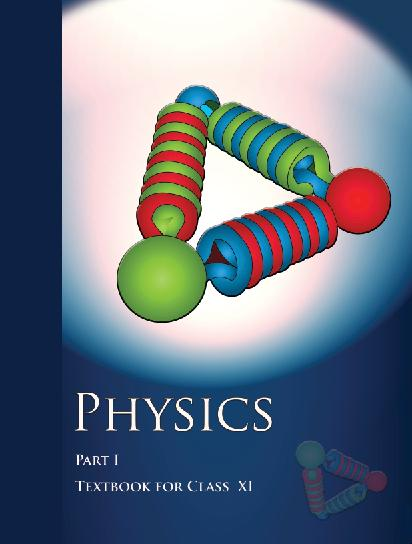## NCERT Class 11 Physics Chapter wise Solutions

• 1 – Physical World
• 2 – Units and Measurements
• 3 – Motion in a Straight line
• 4 – Motion in a Plane
• 5 – Laws of Motion
• 6 – Work, Energy and Power
• 7 – System of Particles and Rotational motion
• 8 – Gravitation
• 9 – Mechanical Properties of Solids
• 10 – Mechanical properties of fluids
• 11 – Thermal Properties of matter
• 12 – Thermodynamics
• 13 – Kinetic Theory
• 14 – Oscillations
• 15 – Waves

## CHAPTER 14 OSCILLATIONS

• 14.1 Introduction
• 14.2 Periodic and oscilatory motions
• 14.3 Simple harmonic motion
• 14.4 Simple harmonic motion and uniform circular motion
• 14.5 Velocity and acceleration in simple harmonic motion
• 14.6 Force law for simple harmonic motion
• 14.7 Energy in simple harmonic motion
• 14.8 Some systems executing SHM
• 14.9 Damped simple harmonic motion
• 14.10 Forced oscillations and resonance

## NCERT Solutions For Class 11 Physics Oscillations

1. Which of the following examples represent periodic motion?

(a) A swimmer completing one (return) trip from one bank of a river to the other and back.

(b) A freely suspended bar magnet displaced from its N-S direction and released.

(c) A hydrogen molecule rotating about its center of mass.

(d) An arrow released from a bow.

2. Which of the following examples represent (nearly) simple harmonic motion and which represent periodic but not simple harmonic motion?

(a) the rotation of earth about its axis.

(b) motion of an oscillating mercury column in a U-tube.

(c) motion of a ball bearing inside a smooth curved bowl, when released from a point slightly above the lower most point.

(d) general vibrations of a polyatomic molecule about its equilibrium position.

3. Figure 14.27 depicts four x-t plots for linear motion of a particle. Which of the plots represent periodic motion? What is the period of motion (in case of periodic motion)?

(a)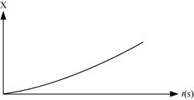(b)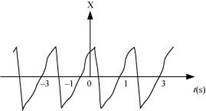(c)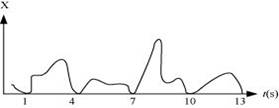(d)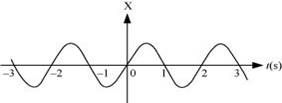4. Which of the following functions of time represent (a) simple harmonic, (b) periodic but not simple harmonic, and (c) non-periodic motion? Give period for each case of periodic motion (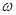is any positive constant):

(a)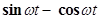(b)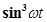(c) 3 cos (π/4 – 2t)

(d) cost + cos 3t + cos 5t

(e) exp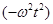(f) 1 +t +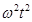5. A particle is in linear simple harmonic motion between two points, A and B, 10 cm apart. Take the direction from A to B as the positive direction and give the signs of velocity, acceleration and force on the particle when it is

(a) at the end A,

(b) at the end B,

(c) at the mid-point of AB going towards A,

(d) at 2 cm away from B going towards A,

(e) at 3 cm away from A going towards B, and

(f) at 4 cm away from B going towards A.

6. Which of the following relationships between the acceleration a and the displacement x of a particle involve simple harmonic motion?

(a) a = 0.7x

(b) a = -200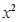(c) a = -10x

(d) a = 100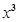7. The motion of a particle executing simple harmonic motion is described by the displacement function,

x (t) = A cos (t +).

If the initial (t = 0) position of the particle is 1 cm and its initial velocity iscm/s, what are its amplitude and initial phase angle? The angular frequency of the particle is π s-1. If instead of the cosine function, we choose the sine function to describe the SHM: x = B sin (t + α), what are the amplitude and initial phase of the particle with the above initial conditions.

8. A spring balance has a scale that reads from 0 to 50 kg. The length of the scale is 20 cm. A body suspended from this balance, when displaced and released, oscillates with a period of 0.6 s. What is the weight of the body?

9. A spring having with a spring constant 1200 N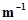is mounted on a horizontal table as shown in Fig. A mass of 3 kg is attached to the free end of the spring. The mass is then pulled sideways to a distance of 2.0 cm and released.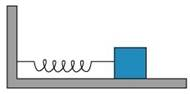Determine (i) the frequency of oscillations, (ii) maximum acceleration of the mass, and (iii) the maximum speed of the mass.

10. In Exercise 14.9, let us take the position of mass when the spring is unstreched as x = 0, and the direction from left to right as the positive direction of x-axis. Give x as a function of time t for the oscillating mass if at the moment we start the stopwatch (t = 0), the mass is

(a) at the mean position,

(b) at the maximum stretched position, and

(c) at the maximum compressed position.

In what way do these functions for SHM differ from each other, in frequency, in amplitude or the initial phase?

11. Figures 14.29 correspond to two circular motions. The radius of the circle, the period of revolution, the initial position, and the sense of revolution (i.e. clockwise or anti-clockwise) are indicated on each figure.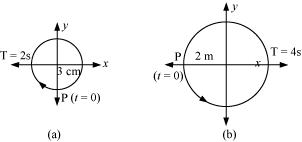Obtain the corresponding simple harmonic motions of the x-projection of the radius vector of the revolving particle P, in each case.

12. Plot the corresponding reference circle for each of the following simple harmonic motions. Indicate the initial (t = 0) position of the particle, the radius of the circle, and the angular speed of the rotating particle. For simplicity, the sense of rotation may be fixed to be anticlockwise in every case: (x is in cm and t is in s).

(a) x = -2 sin (3t + π/3)

(b) x = cos (π/6 – t)

(c) x = 3 sin (2πt + π/4)

(d) x = 2 cos πt

13. Figure 14.30 (a) shows a spring of force constant k clamped rigidly at one end and a mass m attached to its free end. A force F applied at the free end stretches the spring. Figure 14.30 (b) shows the same spring with both ends free and attached to a mass m at either end. Each end of the spring in Fig. 14.30(b) is stretched by the same force F.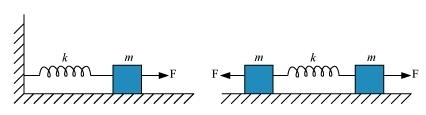(a) What is the maximum extension of the spring in the two cases?

(b) If the mass in Fig. (a) and the two masses in Fig. (b) are released, what is the period of oscillation in each case?

14. The piston in the cylinder head of a locomotive has a stroke (twice the amplitude) of 1.0 m. If the piston moves with simple harmonic motion with an angular frequency of 200 rad/min, what is its maximum speed?

15. The acceleration due to gravity on the surface of moon is 1.7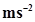. What is the time period of a simple pendulum on the surface of moon if its time period on the surface of earth is 3.5 s? (g on the surface of earth is 9.8)

(a) Time period of a particle in SHM depends on the force constant k and mass m of the particle: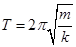. A simple pendulum executes SHM approximately. Why then is the time period of a pendulum independent of the mass of the pendulum?

(b) The motion of a simple pendulum is approximately simple harmonic for small angle oscillations. For larger angles of oscillation, a more involved analysis shows that T is greater than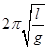. Think of a qualitative argument to appreciate this result.

(c) A man with a wristwatch on his hand falls from the top of a tower. Does the watch give correct time during the free fall?

(d) What is the frequency of oscillation of a simple pendulum mounted in a cabin that is freely falling under gravity?

17. A simple pendulum of length l and having a bob of mass M is suspended in a car. The car is moving on a circular track of radius R with a uniform speed v. If the pendulum makes small oscillations in a radial direction about its equilibrium position, what will be its time period?

18. Cylindrical piece of cork of density of base area A and height h floats in a liquid of density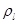. The cork is depressed slightly and then released. Show that the cork oscillates up and down simple harmonically with a period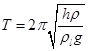Whereis the density of cork. (Ignore damping due to viscosity of the liquid).

19. One end of a U-tube containing mercury is connected to a suction pump and the other end to atmosphere. A small pressure difference is maintained between the two columns. Show that, when the suction pump is removed, the column of mercury in the U-tube executes simple harmonic motion.

20. An air chamber of volume V has a neck area of cross section a into which a ball of mass m just fits and can move up and down without any friction (Fig.14.33). Show that when the ball is pressed down a little and released, it executes SHM. Obtain an expression for the time period of oscillations assuming pressure-volume variations of air to be isothermal [see Fig. 14.33].

21. You are riding in an automobile of mass 3000 kg. Assuming that you are examining the oscillation characteristics of its suspension system. The suspension sags 15 cm when the entire automobile is placed on it. Also, the amplitude of oscillation decreases by 50% during one complete oscillation. Estimate the values of (a) the spring constant k and (b) the damping constant b for the spring and shock absorber system of one wheel, assuming that each wheel supports 750 kg.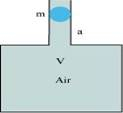22. Show that for a particle in linear SHM the average kinetic energy over a period of oscillation equals the average potential energy over the same period.

23. A circular disc of mass 10 kg is suspended by a wire attached to its centre. The wire is twisted by rotating the disc and released. The period of torsional oscillations is found to be 1.5 s. The radius of the disc is 15 cm. Determine the torsional spring constant of the wire. (Torsional spring constant α is defined by the relation J = -α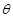¸, where J is the restoring couple and¸ the angle of twist).

24. A body describes simple harmonic motion with amplitude of 5 cm and a period of 0.2 s. Find the acceleration and velocity of the body when the displacement is (a) 5 cm, (b) 3 cm, (c) 0 cm.

25. A mass attached to a spring is free to oscillate, with angular velocity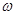, in a horizontal plane without friction or damping. It is pulled to a distance x0 and pushed towards the centre with a velocity v0 at time t = 0. Determine the amplitude of the resulting oscillations in terms of the parameters Ã”°, x0 and v0. [Hint: Start with the equation x = a cos (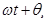) and note that the initial velocity is negative.]

## NCERT Solutions for Class 11 Physics

NCERT Solutions Class 11 Physics PDF (Download) Free from myCBSEguide app and myCBSEguide website. Ncert solution class 11 physics includes text book solutions from both part 1 and part 2. NCERT Solutions for CBSE Class 11 Physics have total 15 chapters. 11 Physics NCERT Solutions in PDF for free Download on our website. Ncert physics class 11 solutions PDF and physics ncert class 11 PDF solutions with latest modifications and as per the latest CBSE syllabus are only available in myCBSEguide

## CBSE app for Class 11

To download NCERT Solutions for class 11 Physics, Chemistry, Biology, History, Political Science, Economics, Geography, Computer Science, Home Science, Accountancy, Business Studies and Home Science; do check myCBSEguide app or website. myCBSEguide provides sample papers with solution, test papers for chapter-wise practice, NCERT solutions, NCERT Exemplar solutions, quick revision notes for ready reference, CBSE guess papers and CBSE important question papers. Sample Paper all are made available through the best app for CBSE students and myCBSEguide website